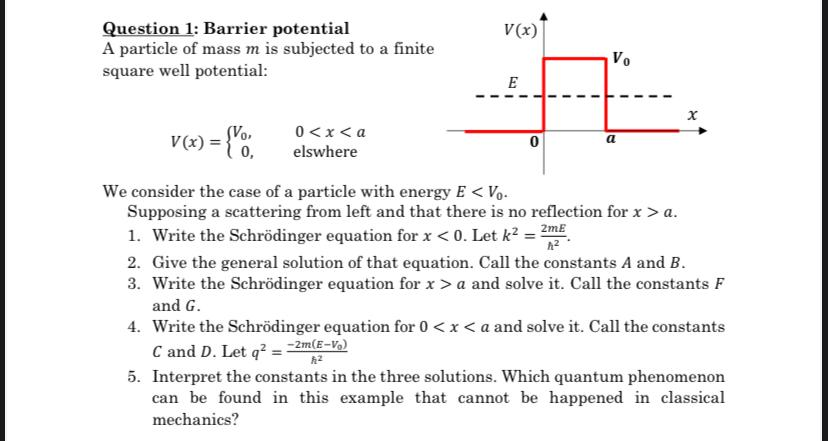# (Solved): Question 1: Barrier potential A particle of mass $$m$$ is subjected to a finite square well pote ...Question 1: Barrier potential A particle of mass $$m$$ is subjected to a finite square well potential: \[ V(x)=\left\{\begin{array}{cc} V_{0}, & 0a \). 1. Write the Schrödinger equation for $$x<0$$. Let $$k^{2}=\frac{2 m E}{\hbar^{2}}$$. 2. Give the general solution of that equation. Call the constants $$A$$ and $$B$$. 3. Write the Schrödinger equation for $$x>a$$ and solve it. Call the constants $$F$$ and $$G$$. 4. Write the Schrödinger equation for \( 0

We have an Answer from Expert